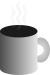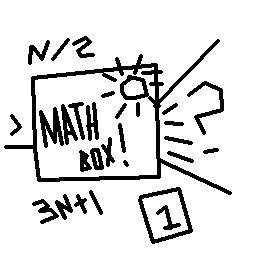#Coffee SpaceListen:

## Collatz ConjecturePreview Image

### Problem Definition

There are two basic rules:

1. If even, `N = N / 2`
2. If odd, `N = 3N + 1`

Iterate down until one is reached.

### Questions

There are a few questions that arise from this:

• Can we predict how many times it would take any given number to roll down to one?
• Are there any cases where it doesn't ever reach one?

### Video

Below is a video that should hopefully explore these ideas and explain the overall concept.

### Code

Here is a link to the GitHub repository so that you can compile and run this experiment yourself on a Unix computer. Some work may be required to get it to run on another Operating System as the code makes use of the GNU Compiler `unsigned __128` type.

### Problems Writing The Code

Following is an explanation of the problems when writing the code. If I was a better human being, there would be tests to make sure this code all runs correctly - alas I am a monster and did not write any tests for this code. Of course, that causes some problems...

#### Converting User Input

When first running the program, I realised that `unsigned long long` wasn't going to cut it, at a measly 64 bits. We were going to need a new conversion function if this is going to work correctly...

We have to take a number from the command line and convert this to `unsigned __int128`. Unfortunately, this doesn't already exist, meaning we have to write our own.

```0001 /**
0002  * charArrTou128()
0003  *
0004  * Converts char* to unsigned __int128 via a manual conversion.
0005  *
0006  * NOTE: This method fails silently.
0007  *
0008  * @param s The string to be converted.
0009  * @return The converted number.
0010  **/
0011 static unsigned __int128 charArrTou128(char* s){
0012   unsigned __int128 r = 0;
0013   int x = 0;
0014   while(s[x] != '\0'){
0015     unsigned __int128 o = r;
0016     r *= 10;
0017     r += (int)(s[x]) - 48;
0018     if(r <= o){
0019       /* Overflow detected */
0020       throw std::overflow_error("string too large for unsigned __int128 conversion");
0021     }
0022     ++x;
0023   }
0024   return r;
0025 }```

Notice the overflow detection on the conversion. This is the following:

```0026 if(r <= o){
0027   /* Overflow detected */
0028   throw std::overflow_error("string too large for unsigned __int128 conversion");
0029 }```

We're basically saying: "If we add something - it should be larger if all inputs are positive and the addition is non-zero?". This is the equivalent of:

```0030 ( (a * 10) + b ) > a
0031 Where:
0032   a < 2^128
0033   b < 10```

The multiplcation of 10 means we should 100% see a larger number. The only case we don't is where we've suffered an overflow error.

#### Converting To `std::string`

Next problem: How can we validate that we converted our number correctly? Ah, of course, simply print it and visually check it. Problem. `std` also doesn't support printing numbers this large - of course. Time to write another function.

```0034 /**
0035  * u128ToCharArr()
0036  *
0037  * Converts unsigned __int128 to char* via a manual conversion.
0038  *
0039  * @param n The number to be converted.
0040  * @return The converted string.
0041  **/
0042 static std::string u128ToCharArr(unsigned __int128 n){
0043   if(n > 0){
0044     /* TODO: Increase the efficiency of this loop. */
0045     std::string s = "";
0046     while(n > 0){
0047       s.insert(0, 1, (char)(((int)'0') + (n % 10)));
0048       n /= 10;
0049     }
0050     return s;
0051   }else{
0052     return "0";
0053   }
0054 }```

#### `3N + 1` Overflow In Main Loop

It's possible that out `3N + 1` calculation may bring us over the limit, after all some of these may jump considerably for a while.

For this reason, we check before we do a that a number is less than or equal to the largest possible starting number, that produces a number that doesn't overflow after the calculation is complete.

```0055 /* Check that n < (((2 ^ 128) - 1) - 1) / 3 for potential calculation size */
0056 if(n <= U128_CALC_MAX){```

### Conclusion

Still waiting on some computing power to run the problem, but overall the program looks fine. Analysing the results may be much harder than the computation. Some ideas to solve this:

• Use a GA to come up with a simplisitic rule set to predict the numbers. The upside is that it will be very clear what the rule is, although it may take a very long time to run.
• Use a neural-network/deep-learning setup to figuer out the relationship. It's a very capable system, but getting the rules out of the network may not be so easy. It's also hard to figure out exactly how to set up the network to optimally tackle the problem and how to prevent over-learning of the problem.